Next: An Example of Cyclical Up: Supporting Theorems Previous: Sequential Software Engineering Methodology

## Cyclical Software Engineering Methodology

In this section, a family of theorems are presented that pertain to the cyclical software engineering methodology. The cyclical software engineering methodology will find solutions in both static and dynamic environments but not in dynamic environments that have the non-monotonic property. If a solution exists, and the cyclical software engineering methodology finds the solution, the best case performance is O(1), the worst case performance is O(N), while the average case performance is O(N) 5.4 where N is the total number of steps in the environment.

Theorem 3 (Cyclical: Static Complete)   A cyclical software engineering methodology is static complete.

Let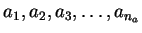be the na steps leading to the possible analysis A with a1 being the initial problem statement. Let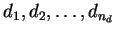be the nd steps leading to the possible design D. Let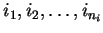be the ni steps leading to the possible implementation I. Let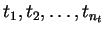be the nt steps leading to the possible testing T with tnt being the final system acceptance test. Though this static environment includes the same steps as in the other sections, these steps are not necessarily taken in the same sequence as those established in the other sections but are the sequence taken by a cyclical methodology. A cyclical methodology has a simple selection process which defines this order. Define a static environment that consists of the four planes of analysis, design, implementation, and testing with each lower plane being a refinement of the higher planes. Define the solution as the tree, or in a more general sense, the directed acyclic graph, of all steps from all four planes used in the final system. This solution can be represented as a sequence of steps. See Figure 5.5 on pagefor a visual representation of the environment. See Figure 5.12 on pagefor a visual guide of the reasoning. The numbers associated with the steps represent the order visited by the methodology.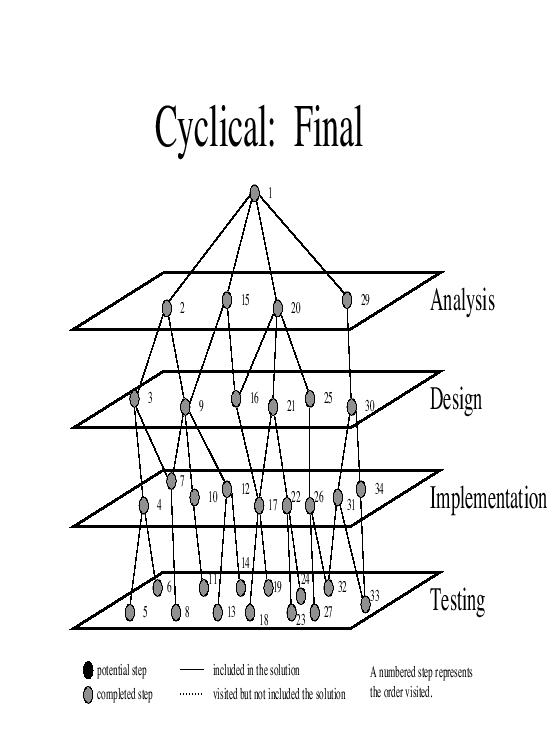Proof 3.1

A cyclic methodology leads to this sequence of steps.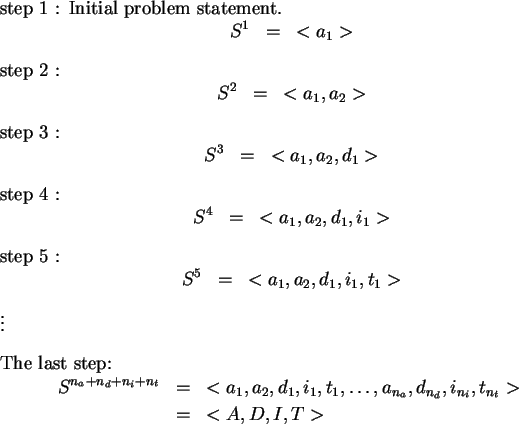The last step is the system acceptance test. Thus a cyclical methodology is static complete.

These steps are generated in an iterative fashion and eventually exhaust the static environment.

If the environment is static, then all steps in the environment are known before the cyclical methodology begins. The cyclical methodology discovers steps in an iterative fashion in the analysis plane, the design plane, the implementation plane, and the testing plane. This iterative discovery of steps from all four planes continues until the environment was exhausted.

Corollary 3.1 (Cyclical: Best Case)   The best case performance of the cyclical software engineering methodology is O(1).

Proof 3.1.1

Let the environment consist of na analysis steps, nd design steps, ni implementation steps, and nt testing steps. Let the correct analysis step be the very first step a1. Let the correct design step be the very first step d1. Let the correct implementation step be the very first step i1. Let the system acceptance test be the very first step t1. To satisfy the problem statement would require one analysis, one design, one implementation, and one testing step. In this environment, the cyclical software engineering methodology will require only four steps. Thus the performance is O(1).

Corollary 3.2 (Cyclical: Worst Case)   The worst case performance of the cyclical software engineering methodology is O(N) where N is the size of the environment.

Proof 3.2.1   Let the environment consist of na analysis steps, nd design steps, ni implementation steps, and nt testing steps. Let the system acceptance test be the very last step tn<<1893>>t. The number of steps to find a solution is na + nd + ni + nt which is O(N).

Corollary 3.3 (Cyclical: Average Case)   The average case performance of the cyclical software engineering methodology is O(N) 5.2 where N is the size of the space.

Proof 3.3.1   Let the environment consist of na analysis steps, nd design steps, ni implementation steps, and nt testing steps. On average, the system acceptance would be in the middle of the testing plane tn<<1902>>t/2. On average, the cyclical software engineering methodology would only have to discover half of the analysis, design, implementation, and testing steps. That is to say, half the time the cyclical software engineering methodology discovers less than half of the space to find a solution and half the time the cyclical software engineering methodology discovers more than half of the space to find a solution. The number of steps to find a solution is (na)/2 + (nd)/2 + (ni)/2 + (nt)/2 which is N/2 steps. Thus, the performance is O(N). 5.3

Corollary 3.4 (Cyclical: Dynamic Complete)   A cyclical software engineering methodology is dynamic complete.

Proof 3.4.1   A cyclical methodology allows for the introduction of new information at every cycle and the removal of information that is no longer needed. Thus, for a dynamic environment, a cyclical software engineering methodology will eventually find the solution.

Corollary 3.5 (Cyclical: Non-monotonic Incomplete)   A cyclical software engineering methodology is non-monotonic incomplete.

Proof 3.5.1

The cyclical software engineering methodology has no mechanism to manage a non-monotonic step. Consider a dynamic environment with two highly conflicting requirements and their associated design, implementation, and testing steps. The cyclical software engineering methodology would pick one requirement and build the associated system. The cyclical software engineering methodology, because there is no mechanism to manage a non-monotonic step, then picks the second conflicting requirement. The first solution is negated in order to build a second system. The cyclical software engineering methodology oscillates between these two systems with no convergence to a common solution. The final system acceptance test fails because one of the conflicting requirements can never be accomplished. Thus, the cyclical software engineering methodology is incomplete for a non-monotonic environment.Next: An Example of Cyclical Up: Supporting Theorems Previous: Sequential Software Engineering Methodology
Ronald LeRoi Burback
1998-12-14